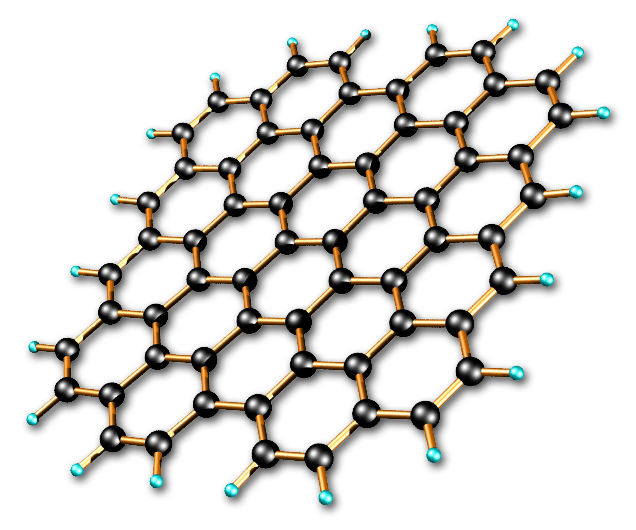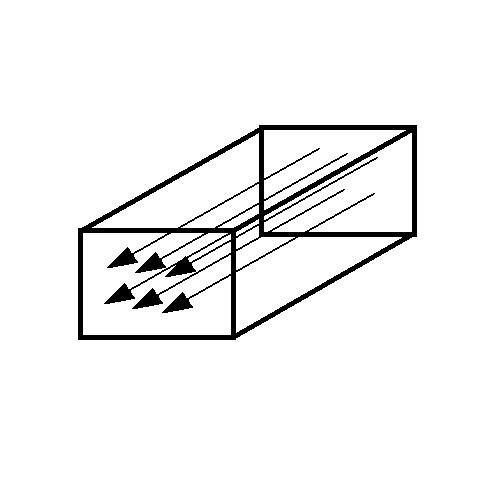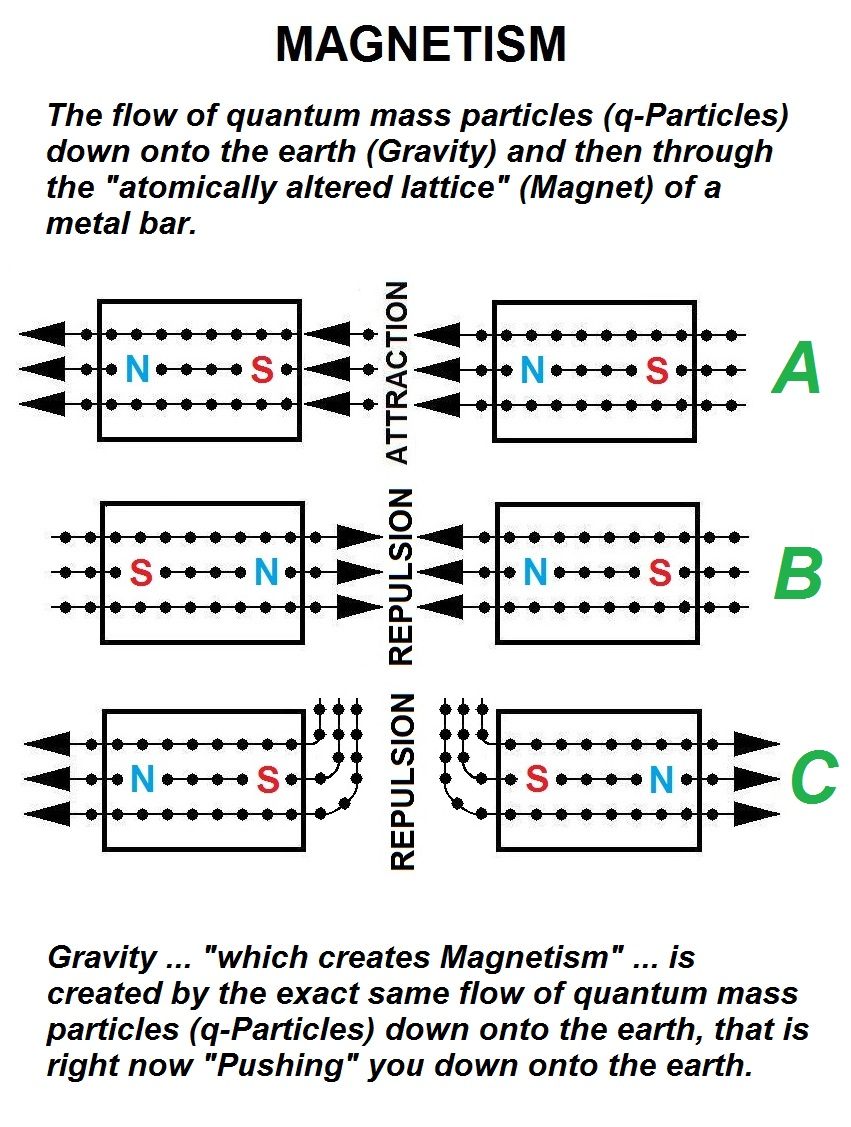E i n s t e i n M a g n e t i s m . c o m

The  Unification  of  Gravity  and  Magnetism

( mathematically proven on  EinsteinElectricity.com )

After thousands of years ... man finally knows "exactly" what causes

The Force of Magnetism.

Gravity is the result of the "Flow" of Quantum-Mass Particles (q-Particles) into the earth and that flow is right now "Pushing" you down onto the earth.  And as that quantum mass moves into the earth it creates the earth's Magnetic Field just like the magnetic field around any common magnet.

When a piece of metal is turned it into a magnet via the use of electricity (changing its atomic lattice structure)the atomic structure is rearranged so that the flow of q-Particles passes through the structure in only "one direction" or at least much more easily in that one direction.This is why an object becomes Magnetized, just a one-way tunnel for q-Particles passing through it.  All of the quantum mass around the metal bar (like water at the bottom of the ocean) is wanting to enter the bar via the pressure of Gravity.  Quantum mass upon all of its surfaces is forcing its way into the metal bar and due to the rearrangement of the atomic lattice, the flow now occurs more easily in only one direction.  The bar has now become a very simple quantum-particle-flow gun.

This flow of q-Particles through the metal bar

is the very definition of Magnetism.The flow of quantum-mass (Gravity) that surrounds the earth now enters all of the surfaces of the two bars and then flows through the bars much easier in one direction which then results in the bars either;

A -   being attracted towards each other via the same Bernoulli effect as an atomizer that draws up liquid.

B -  repelling each other as the flowing q-Particles cannot pass through themselves.

C -  repelling each other as the flowing q-Particles gather at the entrance of the two magnets and once again do not allow passage through themselves.

Magnetism is the flow of q-Particles through the altered lattice of a piece of mass and that flow is caused by the same flow of Gravity.  (The very reason why the velocity of Magnetism and the velocity of Gravity is identical.)

Quantum-Mass "Flow" is the root cause of Gravity.

And Gravity is the  root cause of Magnetism.

Heated air does not rise because it is hotter, it rises because it has a greater (A) value.  Any particle of Mass with a greater A value has a higher temperature because Temperature = AxZDue to the fact that quantum-mass Density is equal to m/A3Z6 we can see the relationship between Density and the Acceleration of Gravity and that the hot air is really being "pushed less" downward by Gravity due to the heated particle's lesser Density.  The quantum-mass (Gravity) moving downward through hotter air moves more easily through it and therefore does not push as hard upon it and therefore it rises due to the colder (more dense) air around it being pushed down more readily.

MAGNETISM

The accepted equation for magnetism is;

F = q(v x B)

F  =  Magnetic Force  (F = Electrical-Mass x Acceleration) (F = Newton) (mA)

q  =  Electric charge of the particle  (q = Coulombs) (mA)

v  =  Velocity vector of particle  (v = Meters / Second) (AZ)

B  =  Magnetic field  (B = Weber / Meter2) (Teslas) (1/AZ)

The above is in complete unity with the one true Energy equation derived from  EinsteinElectricity.com

E = mA2Z2

and all its variables;

 Electricity = m(AZ)2 Quantum-mass = m Acceleration = A Time = Z Joule = mA2Z2 Watt = mA2Z Coulomb = mA Volt = AZ2 Amp = mA/Z Weber = AZ3 Farad = m/Z2 Ohm = Z3/m Tesla = 1/AZ Siemen = m/Z3 El. Field =  1/1 ( Volt / Meter ) Henry = Z4/m

The Unification of Gravity and Magnetism

discovered by  AZ

See the complete and amazing story at